# Model theory of the real exponential function

(diff) ← Older revision | Latest revision (diff) | Newer revision → (diff)
Jump to: navigation, search

A branch of model theory studying the elementary theory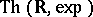of the ordered fieldof real numbers with the real exponential function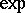(cf. Exponential function, real). It is motivated by Tarski's question [a7], p. 45, whetheris decidable.

A. Wilkie showed in [a8] thatis model complete. Combining this with Khovanskii's finiteness theorem [a5], it follows that this theory is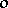-minimal. In fact, Wilkie first studies expansions (cf. Structure) ofby a Pfaffian chain of functions (see also [a2]): Fix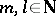and an open setcontaining the closed unit box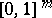. A Pfaffian chain of functions onis a sequence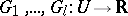of analytic functions (cf. Analytic function) for which there exist polynomials(for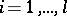;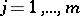) such thatfor all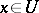. Wilkie shows that the expansion ofby a Pfaffian chain of functions restricted to the closed unit box has a model-complete theory. In particular, the expansion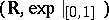ofby the restricted exponential function has a model-complete theory. Wilkie then deduces the model completeness offrom this last result. An alternative proof of the model completeness, and an axiomatization ofover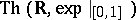, was found by J.P. Ressayre in 1991 (see [a3] for a generalization of Ressayre's result).

In [a6], A. Macintyre and Wilkie show thatis decidable provided that the real version of Schanuel's conjecture (cf. Algebraic independence) is true.

The theorydoes not admit elimination of quantifiers. In fact, an expansion ofby a family of total real-analytic functions (see [a1]) admits elimination of quantifiers if and only if each function is semi-algebraic, i.e., has a semi-algebraic graph (cf. Semi-algebraic set). However, letdenote the family of restricted real-analytic functions, i.e., functions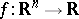, for all, which are given on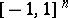by a power series converging on a neighbourhood ofand are set equal tooutside of. It is shown in [a3] that the expansion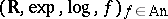admits elimination of quantifiers. The authors also give a complete axiomatization of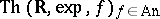, and establish that it is-minimal. In [a4] they construct a model of this theory which is not Archimedean and use it to solve a problem raised by G.H. Hardy: they show that the compositional inverse of the function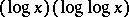is not asymptotic at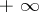to a composition of semi-algebraic functions,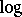and.